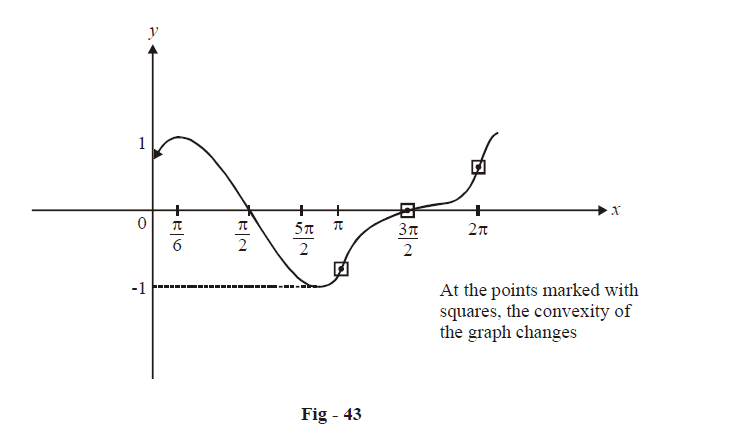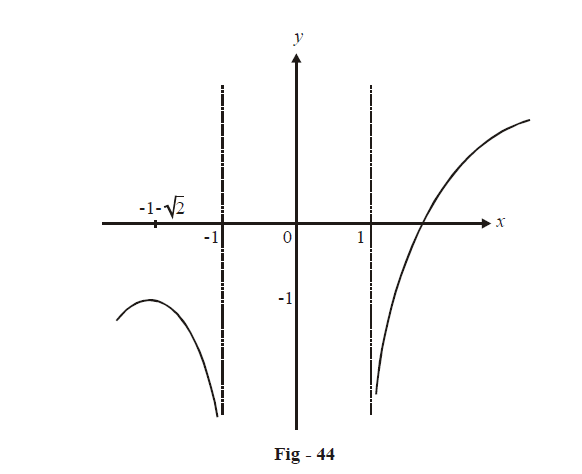# Examples on Graphing Using Derivatives Set 3

Go back to  'Applications of Derivatives'

Example - 39

Plot the graph of \begin{align}f\left( x \right) = \frac{1}{2}\sin 2x + \cos x.\end{align}

Solution:   *      The domain of (x) is $$\mathbb{R}$$.

*      $$f\left( x \right)$$ is periodic with period $$2\pi$$ and therefore we need to analyze it only in $$[0,2\pi ]$$

*      $$f\left( x \right)$$  is continuous and differentiable on $$\mathbb{R}$$

*      There are no asymptotes to f(x)

*      $$f'\left( x \right) = \cos 2x - \sin x$$

\begin{align} &= 1 - 2{\sin ^2}x - \sin x\\\\ &= \left( {1 + \sin x} \right)\left( {1 - 2\sin x} \right)\end{align}

This is 0 in $$\left[ 0,2\pi \right]$$ when

\begin{align}x = \frac{\pi }{6},\frac{{5\pi }}{6},\frac{{3\pi }}{2}\end{align}

$$f\left( x \right) = - 2\sin 2x - \cos x$$

Now, \begin{align}\;\; f''\left( {\frac{\pi }{6}} \right)< 0,\,\,f\left( {\frac{{5\pi }}{6}} \right) > 0\end{align} and \begin{align}f\left( {\frac{{3\pi }}{2}} \right) = 0\end{align}

\begin{align} \Rightarrow \quad x = \pi /6\end{align} is a local maximum for \begin{align}f\left( x \right);f\left( {\frac{\pi }{6}} \right) = \frac{{3\sqrt 3 }}{4}\end{align}

\begin{align} x = \frac{{5\pi }}{6}\end{align} is a local minimum for \begin{align} f\left( x \right);f\left( {\frac{{5\pi }}{6}} \right) = \frac{{ - 3\sqrt 3 }}{4}\end{align}

\begin{align} x = \frac{{3\pi }}{2}\end{align} is a point of inflexion; \begin{align} f\left( {\frac{{3\pi }}{2}} \right) = 0.\end{align}

We now need to analyze to sign of $$f''\left( x \right).$$

\begin{align} &f''\left( x \right) = - 2\sin 2x - \cos x\\\\&\qquad= - 4\sin x\cos x - \cos x\\\\& \qquad= - \cos x\left( {1 + 4\sin x} \right)\end{align}

This is 0 in $$[0,2\pi ]$$ when

$x = \frac{\pi }{2},\pi + {\sin ^{ - 1}}\frac{1}{4},\,\frac{{3\pi }}{2},2\pi - {\sin ^{ - 1}}\frac{1}{4}$

We see that (x) will change its convexity at four different points.

$\Rightarrow \quad f'' \left( x \right) > 0\,\,\forall x \in \left( {\frac{\pi }{2},\pi + {{\sin }^{ - 1}}\frac{1}{4}} \right) \cup \left( {\frac{{3\pi }}{2},2\pi - {{\sin }^{ - 1}}\frac{1}{4}} \right)$

so that (x) is concave upwards in these intervals

$\Rightarrow \quad f''\left( x \right) < 0\,\;\;\forall x \in \left( {0,\frac{\pi }{2}} \right) \cup \left( {\pi + {{\sin }^{ - 1}}\frac{1}{4},\frac{{3\pi }}{2}} \right) \cup \left( {2\pi - {{\sin }^{ - 1}}\frac{1}{4},2\pi } \right)$

so that (x) is concave downwards in these intervals.

\begin{align}* \qquad f\left( 0 \right) = 1,f\left( {\frac{\pi }{2}} \right) = 0,\,\,f\left( {2\pi } \right) = 1\end{align}

The graph has been plotted below for $$\left[ 0,2\pi \right]$$Example - 40

Plot the graph of $$y = x + \ln \left( {{x^2} - 1} \right)$$.

Solution:

*         The Domain is given by

\begin{align} &\qquad\quad{x^2} - 1 > 0 \\ & \Rightarrow \quad \,D = \mathbb{R}\backslash [ - 1,\,\,1] \\ \end{align}

*         $$f\left( x \right)$$ is continuous and differentiable on D

*         $$\mathop {\lim }\limits_{x \to {1^ + }} y = - \infty \,\,;\,\,\,\mathop {\lim }\limits_{x \to - {1^ - }} y = - \infty$$

$$\Rightarrow \, x = \pm 1$$ are vertical asymptotes to the curve.

Verify that the graph has no other asymptotes

\begin{align}&* \qquad y'=1+\frac{2x}{{{x}^{2}}-1} \\\\ & \qquad \quad y'=0\;\text{when}\,\,{{x}^{2}}+2x-1=0 \\\\ & \qquad\qquad\quad\quad\Rightarrow \quad x=-1\pm \sqrt{2} \\\\ &\qquad\qquad\qquad\quad \quad\;\; x=-1+\sqrt{2}\,\,\,\text{does}\,\text{not}\,\text{belong}\,\text{to}\,\text{D;} \\\\ & \qquad\quad \qquad \qquad \quad\;\; x=-1-\sqrt{2}\,\,\text{is}\,\text{an}\,\text{extremum}\,\text{point} \\\\ & \qquad \quad\quad \quad\;\; \Rightarrow \quad y''=-\frac{2\left( {{x}^{2}}+1 \right)}{{{\left( {{x}^{2}}-1 \right)}^{2}}}<0\,\,\,\,\,\forall \,x \\ \end{align}

$$\Rightarrow$$ The curve is always concave downwards so that $$x = - 1 - \sqrt 2$$ is a point of local maximum.

*  $$\mathop {\lim }\limits_{x \to + \infty } y = \infty \,\,\,;\,\,\mathop {\lim }\limits_{x \to - \infty } y = - \infty$$

Based on this data, the graph can be plotted as shown below:## TRY YOURSELF - VI

Q. 1     Plot the graphs of the following functions by completely analysing their corresponding equations    (as done in class; this means that you have to analyse their limits at different points as well as their first and second derivatives in different intervals):

 (i) \begin{align}\quad y = \frac{1}{{1 - {x^2}}}\end{align} (ii)\begin{align}\quad y = \frac{x}{{{x^2} - 1}}\end{align} (iii) \begin{align}\quad y = \frac{1}{{\left( {x - 1} \right)\left( {x - 2} \right)\left( {x - 3} \right)}}\end{align} (iv)\begin{align}\quad y = \frac{1}{x} + 4{x^2}\end{align} (v)\begin{align}\quad y = {x^2} + \frac{1}{{{x^2}}}\end{align} (vi)\begin{align}\quad y = \frac{{{x^3}}}{{3 - {x^2}}}\end{align} (vii)\begin{align}\quad y = \frac{{{x^3}}}{{2{{\left( {x + 1} \right)}^2}}}\end{align} (viii)\begin{align}\quad y = \frac{{{x^3}}}{{x - 1}}\end{align} (ix)\begin{align}\quad y = \frac{{{x^4}}}{{{x^3} - 1}}\end{align} (x)\begin{align}\quad y = \frac{{{{\left( {x - 1} \right)}^2}}}{{{{\left( {x + 1} \right)}^3}}}\end{align} (xi)\begin{align}\quad y = \frac{{\left( {{x^2} - 1} \right)\left( {x - 2} \right)}}{x}\end{align} (xii)\begin{align}\quad y = \frac{x}{{{e^x}}}\end{align} (xiii)\begin{align}\quad y = {x^2}{e^{ - x}}\end{align} (xiv)\begin{align}\quad y = \frac{{{e^x}}}{x}\end{align} $$(xv)\quad y = x - \ln \left( {x + 1} \right)$$ $$(xvi) \quad y = \ln \left( {{x^2} + 1} \right)$$ (xvii)\begin{align}\quad y = {x^2}{e^{ - {x^2}}}\end{align} $$(xviii) \quad y = {x^3}{e^{ - x}}$$ (xix)\begin{align}\quad y = x{e^{\frac{{ - {x^2}}}{2}}}\end{align} (xx)\begin{align}\quad y = \frac{1}{{{e^x} - 1}}\end{align} (xxi)\begin{align}\quad y = x + \frac{{\ln x}}{x}\end{align} (xxii)\begin{align}\quad y = {\left( {1 + \frac{1}{x}} \right)^x}\end{align} (xxiii) \begin{align}\quad y = x + \sin x\end{align} $$(xxiv)\quad y = \ln \cos x$$ $$(xxv) \quad y = x\sin x$$ $$(xxvi)\quad y = x - 2{\tan ^{ - 1}}x$$ $$(xxvii)\quad {y^2} = {x^3} - x$$ $$(xxviii)\quad {y^2} = {x^3} + 1$$ $$(xxix)\quad {y^2} = {x^2} - {x^4}$$ $$(xxx)\quad y = \cos x - \ln \cos x$$

Q. 2     Find the asymptotes of the following curves and then graph them:

 (i)\begin{align}\quad \frac{{{x^2}}}{{{a^2}}} - \frac{{{y^2}}}{{{b^2}}} = 1\end{align} $$(ii)\quad xy = a$$ (iii)\begin{align} \quad y = \frac{1}{{{x^2} - 4x + 5}}\end{align} $$(iv)\quad {y^3} = {a^3} - {x^3}$$ $$(v)\quad y = x\,{e^x}$$ (vi) \begin{align} \quad y = x\,{e^{\frac{2}{x}}} + 1 \end{align} $$(vii)\quad {\left( {y + x + 1} \right)^2} = {x^2} + 1$$ $$(viii)\quad x{y^2} + {x^2}y = {a^3}$$ $$(ix)\quad {y^3} = 6{x^2} + {x^3}$$ (x)\begin{align} \quad y = 2x + {\tan ^{ - 1}}\frac{x}{2}\end{align}